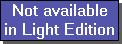The SDL Component Suite is an industry leading collection of components supporting scientific and engineering computing. Please visit the SDL Web site for more information....## PenalizedCubicSplineUnit: SDL_math2 Class: none Declaration: procedure PenalizedCubicSpline (n1, n2, smooth: integer; x, y, dy: array of double; var a,b,c,d: array of double);

The procedure PenalizedCubicSpline calculates a penalized cubic spline for n2-n1+1 data points as it has been published by C.H.Reinsch [Num.Math. 10 (1967) 177]. The parameters n1 and n2 are the lower and the upper index of the range of data to be included in the calculation. The x- and y-values of the data are stored in the arrays x and y. The array dy contains the relative importance of the corresponding points. Please note that the following inequality must hold true:
n2 > n1 > 0,

which means that you cannot use the first point of the data array (x|y) for calculating the spline function.

The parameter smooth controls the extent of smoothing and must be greater or equal to zero. A zero value means no smoothing. Please note that the parameter smooth and the array dy depend on each other. Recommended values for dy are the estimated standard deviations of the ordinate for a given x-value. In this case useful values for the smooth parameter lie within the interval n +/- sqrt(2n), with n being the number data points used for the approximation.

On return the variable arrays a, b, c, and d contain the smoothed spline parameters. In order to calculate the spline result for a particular argument xx one first has to calculate the difference between xx and the next lower value of the array x (index ix). Then the function result is given by

f(xx) = ((d[ix]*h + c[ix])*h + b[ix])*h + a[ix]

 Hint 1: The values of the array x must be strictly increasing. If two x-values are equal or not in increasing order, an exception is thrown.

 Hint 2: A much easier to use smoothed spline interpolation is the method SmoothedSpline of the class TCurveFit. This method is based on the procedure PenalizedCubicSpline making a few simplifying assumptions.

Last Update: 2012-Oct-20